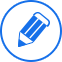#패밀리사이트 보기 전체메뉴# 이전 화면으로 돌아가기

• 사이트
• 인강
• 학원
• 어학·유학
• 국가고시
• 자격증·학위·전문직
• 취업·편입·전문대학원

#### 취업·편입·전문대학원

• 어학·유학
• 국가고시
• 자격증·학위·전문직
• 취업·편입·전문대학원

#### 취업·편입·전문대학원

• 어학·유학
• 국가고시
• 자격증·학위·전문직
• 취업·편입·전문대학원

#### 취업·편입·전문대학원• 취소신고

## 유학 Q&A

전자공학과분 계신가요.
goom | 2005.10.04 | 조회 318
This problem considers the use of pre-filtering to reduce the high-frequency content of a signal prior to sampling it. By pre-filtering, we can reduce or eliminate the aliasing introduced by sampling. To keep the problem simple, we are considering 1D sampling of audio (temporal) signals rather than 2D sampling of image (spatial) signals. Thus, in the Fourier domain the units are Hz (temporal cycles/second). Digital telephone systems sample audio signals at a rate of 8kHz. a) What is the highest audio frequency that can be sampled by a telephone system without aliasing? Humans can hear frequencies up to approximately 20 kHz. For the sake of simplicity, assume that the audio signal that we are sampling has a flat frequency spectrum from 0 to 20KHz. To avoid aliasing when we sample this signal, we are going to first pre-filter it with an analog filter to reduce the amount of energy in the signal above the Nyquist frequency. Our filter operates in the time domain (equivalent to the spatial domain for images). Our filter is implemented as convolution with a Gaussian in the time domain. b) Express the filter mathematically in both the time domain and the frequency domain. i.e. what is h(t) and what is H( ω)? The width of h(t) should be expressed in terms of a standard deviation σ. Because the Guassian never becomes exactly zero, we cannot use a guassian as a perfect pre-filter. But, if we choose the width of the Gaussian well it can be a good filter. Suppose that we want to insure that 98% of the energy in the sampled signal is not aliased (i.e. 98% of the energy in the sampled signal comes from frequencies that were below the Nyquist frequency in the original audio signal). c) What width of Guassian should we use? (Hint, you are going to have to consider area under the curve H( ω)) d) With the filter from part C, what fraction of the original signal energy below the Nyquist frequency are we discarding? Ideally the answer would be 0%, but the answer is higher than that because the Gaussian is not a perfect low-pass filter. 문제가 너무 어려운데 c,d 문제에 관한 조언 좀 해주실수 있는지요... 감사합니다.
윗   글
TAMU
아랫글
[re] 전자공학과분 계신가요.